Scan QR code or get instant email to install app

Question:

# A circle's area of 280 meters is reduced by 60%. What is the ratio of the reduced radius to the original radius?

A 0.63
explanation

1212When the original area is reduced by 60%, only 40% remains. Find the reduced area by multiplying the original area by 40%: 280 \u00D7 0.4 = 112. Solve for the radius of the original circle: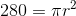, so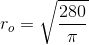. Solve for the radius of the reduced circle: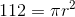, so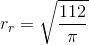. When evaluating the ratio of the reduced radius to the original radius, the π in both denominators will cancel to leave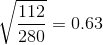.